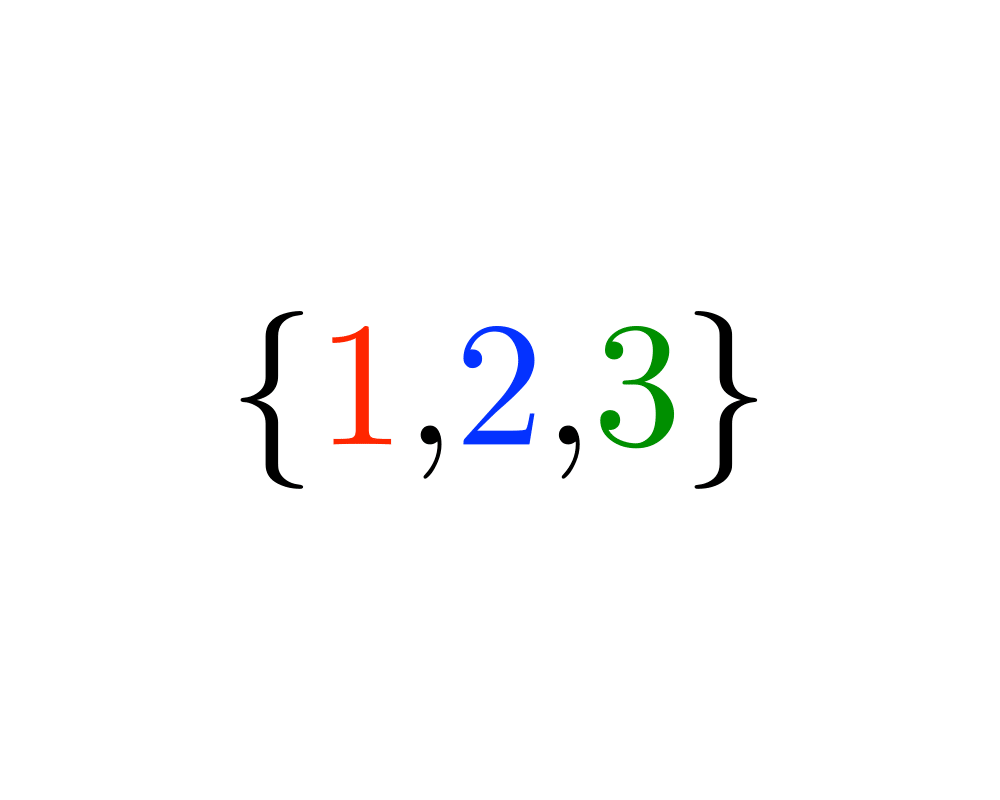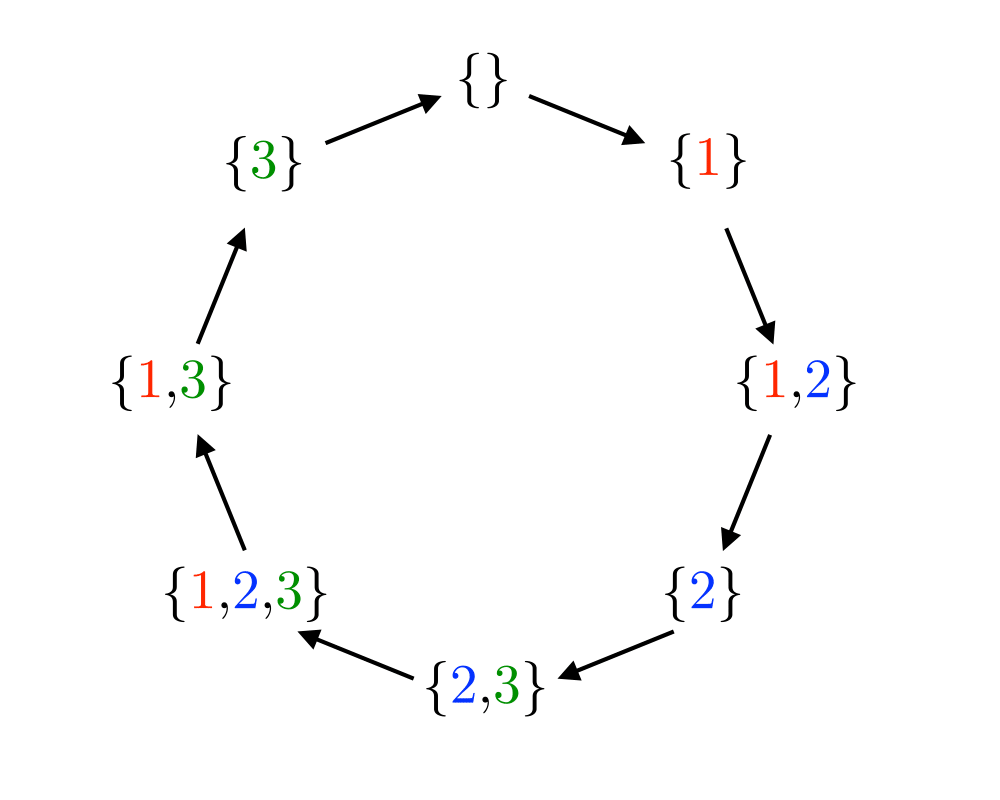# Generating SubsetsInputOutput

Input Description: An integer $$n$$.
Problem: Generate (1) all, or (2) a random, or (3) the next subset of the integers $$1$$ to $$n$$.

Excerpt from The Algorithm Design Manual: A subset describes a selection of objects, where the order among them does not matter. Many of the algorithmic problems in this catalog seek the best subset of a group of things: vertex cover seeks the smallest subset of vertices to touch each edge in a graph; knapsack seeks the most profitable subset of items of bounded total size; and set packing seeks the smallest subset of subsets that together cover each item exactly once.

There are $$2n$$ distinct subsets of an $$n$$-element set, including the empty set as well as the set itself. This grows exponentially, but at a considerably smaller rate than the $$n!$$ permutations of $$n$$ items. Indeed, since $$220 =$$ 1,048,576, a brute-force search through all subsets of 20 elements is easily manageable, although by $$n=30$$, $$230 =$$ 1,073,741,824, so you will certainly be pushing things.

Go To Main Page